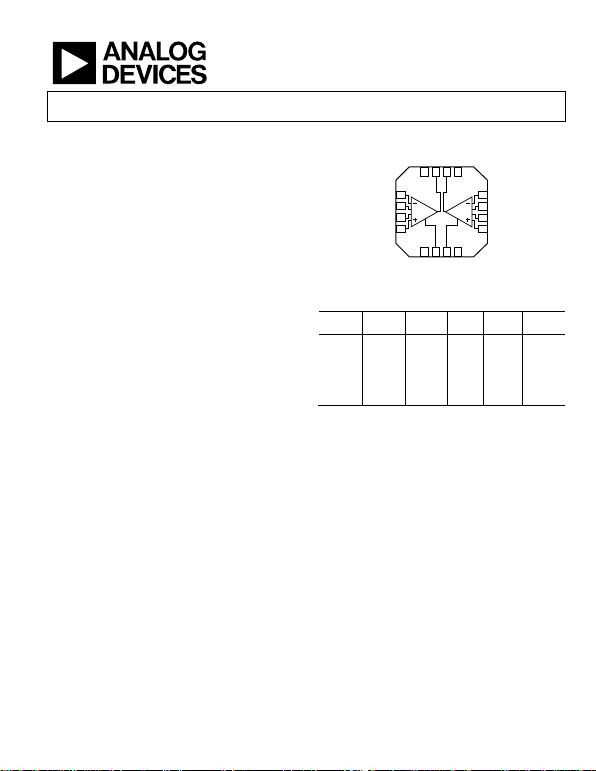Precision, Dual-Channel, JFET Input,
Rail-to-Rail Instrumentation Amplifier
Rev. B
Information furnished by Analog Devices is believed to be accurate and reliable. However, no
responsibility is assumed by Analog Devices for its use, nor for any infringements of patents or other
rights of third parties that may result from its use. Specifications subject to change without notice. No
license is granted by implication or otherwise under any patent or patent rights of Analog Devices.
One Technology Way, P.O. Box 9106, Norwood, MA 02062-9106, U.S.A.
Tel: 781.329.4700 www.analog.com
FEATURES
Two channels in a small 4 mm × 4 mm LFCSP
Custom LFCSP package with hidden paddle
Permits routing and vias underneath package
Allows full bias current performance
Low input currents
10 pA maximum input bias current (B Grade)
0.6 pA maximum input offset current (B Grade)
High CMRR
100 dB CMRR (minimum), G = 10 (B Grade)
90 dB CMRR (minimum) to 10 kHz, G = 10 (B Grade)
Excellent ac specifications and low power
1.5 MHz bandwidth (G = 1)
14 nV/√Hz input noise (1 kHz)
Slew rate: 2 V/μs
750 μA quiescent current per amplifier
Versatility
Rail-to-rail output
Input voltage range to below negative supply rail
4 kV ESD protection
4.5 V to 36 V single supply
±2.25 V to ±18 V dual supply
Gain set with single resistor (G = 1 to 1000)
APPLICATIONS
Medical instrumentation
Precision data acquisition
Transducer interfaces
Differential drives for high resolution input ADCs
Remote sensors
FUNCTIONAL BLOCK DIAGRAM
1
2
3
4
12
11
10
9
5678
13141516
–IN1
R
G1
R
G1
+IN1
–IN2
R
G2
R
G2
+IN2
+V
S
REF1
REF2
–V
S
+V
S
OUT1
OUT2
–V
S
06286-001
Figure 1.
Table 1. In Amps and Difference Amplifiers by Category
High
Perform
Low
Cost
High
Voltage
Mil
Low
Power
Digital
Gain
1 Rail-to-rail output.
GENERAL DESCRIPTION
The AD8224 is the first single-supply, JFET input instrumentation
amplifier available in the space-saving 16-lead, 4 mm × 4 mm
LFCSP. It requires the same board area as a typical single
instrumentation amplifier yet doubles the channel density
and offers a lower cost per channel without compromising
performance.
Designed to meet the needs of high performance, portable
instrumentation, the AD8224 has a minimum common-mode
rejection ratio (CMRR) of 86 dB at dc and a minimum CMRR
of 80 dB at 10 kHz for G = 1. Maximum input bias current is
10 pA and typically remains below 300 pA over the entire
industrial temperature range. Despite the JFET inputs, the
AD8224 typically has a noise corner of only 10 Hz.
With the proliferation of mixed-signal processing, the number
of power supplies required in each system has grown. Designed
to alleviate this problem, the AD8224 can operate on a ±18 V
dual supply, as well as on a single +5 V supply. The devices rail-
to-rail output stage maximizes dynamic range on the low
voltage supplies common in portable applications. Its ability to
run on a single 5 V supply eliminates the need for higher
voltage, dual supplies. The AD8224 draws 750 µA of quiescent
current per amplifier, making it ideal for battery powered
devices.
differential output, instrumentation amplifier. Differential
outputs provide high noise immunity, which can be useful when
the output signal must travel through a noisy environment, such
as with remote sensors. The configuration can also be used to
drive differential input ADCs. For a single-channel version, useRev. B | Page 2 of 28
Features .............................................................................................. 1
Applications ....................................................................................... 1
Functional Block Diagram .............................................................. 1
General Description ......................................................................... 1
Revision History ............................................................................... 2
Specifications ..................................................................................... 3
Absolute Maximum Ratings ............................................................ 9
Thermal Resistance ...................................................................... 9
ESD Caution .................................................................................. 9
Pin Configuration and Function Descriptions ........................... 10
Typical Performance Characteristics ........................................... 11
Theory of Operation ...................................................................... 20
Gain Selection ............................................................................. 20
Reference Terminal .................................................................... 21
Layout .......................................................................................... 21
Solder Wash ................................................................................. 22
Input Bias Current Return Path ............................................... 22
Input Protection ......................................................................... 22
RF Interference ........................................................................... 23
Common-Mode Input Voltage Range ..................................... 23
Applications Information .............................................................. 24
Differential Output .................................................................... 24
Driving a Differential Input ADC ............................................ 25
Driving Cabling .......................................................................... 25
Outline Dimensions ....................................................................... 26
Ordering Guide .......................................................................... 27
REVISION HISTORY
5/10—Rev. A to Rev. B
Changes to Features Section............................................................ 1
Changes to Figure 3 and Table 11 ................................................. 10
Package Section and Figure 58 ...................................................... 21
Updated Outline Dimensions ....................................................... 26
Changes to Ordering Guide .......................................................... 27
4/07—Rev. 0 to Rev. A
Changes to Features, General Description, and Figure 1 ............ 1
Changes to Table 2 ............................................................................ 3
Changes to Table 3 and Table 4 ....................................................... 5
Changes to Table 5 ............................................................................ 6
Changes to Table 6 and Table 7 ....................................................... 8
Changes to Figure 2 ........................................................................... 9
Changes to Figure 3 ........................................................................ 10
Inserted Figure 4, Figure 5, and Figure 6; Renumbered
Sequentially ..................................................................................... 11
Changes to Figure 7 ........................................................................ 11
Changes to Figure 20 and Figure 21............................................. 13
Changes to Figure 28 ...................................................................... 15
Changes to Theory of Operation and Figure 55 ........................ 20
Changes to Ordering Guide .......................................................... 26
1/07—Revision 0: Initial Version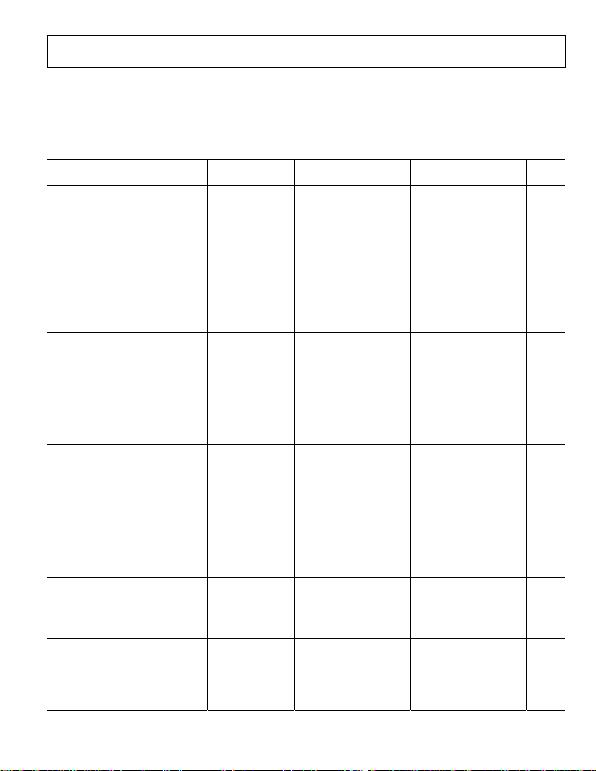Rev. B | Page 3 of 28
SPECIFICATIONS
VS+ = +15 V, VS− = −15 V, VREF = 0 V, TA = 25°C, G = 1, RL = 2 k1, unless otherwise noted. Table 2 displays the specifications for an
individual instrumentation amplifier configured for a single-ended output or dual instrumentation amplifiers configured for differential
outputs as shown in Figure 63.
Table 2. Individual Amplifier in Single-Ended Configuration or Dual Amplifiers in Differential Output Configuration2, VS = ±15 V
Parameter Test Conditions Min Typ Max Min Typ Max Unit
COMMON-MODE REJECTION RATIO (CMRR)
CMRR DC to 60 Hz with
1 kΩ Source Imbalance
VCM = ±10 V
G = 1 78 86 dB
G = 10 94 100 dB
G = 100 94 100 dB
G = 1000 94 100 dB
CMRR at 10 kHz VCM = ±10 V
G = 1 74 80 dB
G = 10 84 90 dB
G = 100 84 90 dB
G = 1000 84 90 dB
NOISE RTI noise =
√(eni2 + (eno/G)2)
Voltage Noise, 1 kHz
Input Voltage Noise, eni V
IN+, VIN− = 0 V 14 14 17 nV/√Hz
Output Voltage Noise, eno V
IN+, VIN− = 0 V 90 90 100 nV/√Hz
RTI, 0.1 Hz to 10 Hz
G = 1 5 5 μV p-p
G = 1000 0.8 0.8 μV p-p
Current Noise f = 1 kHz 1 1 fA/√Hz
VOLTAGE OFFSET RTI VOS =
(VOSI) + (VOSO/G)
Input Offset, VOSI 300 175 μV
Average TC T = −40°C to +85°C 10 5 μV/°C
Output Offset, VOSO 1200 800 μV
Average TC T = −40°C to +85°C 10 5 μV/°C
Offset RTI vs. Supply (PSR) VS = ±5 V to ±15 V
G = 1 86 86 dB
G = 10 96 100 dB
G = 100 96 100 dB
G = 1000 96 100 dB
INPUT CURRENT (PER CHANNEL)
Input Bias Current 25 10 pA
Over Temperature3 T = −40°C to +85°C 300 300 pA
Input Offset Current 2 0.6 pA
Over Temperature3 T = −40°C to +85°C 5 5 pA
REFERENCE INPUT
RIN 40 40
IIN V
IN+, VIN− = 0 V 70 70 μA
Voltage Range −VS +VS −VS +VS V
Gain to Output 1 ±
0.0001
1 ±
0.0001
V/VRev. B | Page 4 of 28
Parameter Test Conditions Min Typ Max Min Typ Max Unit
GAIN G = 1 + (49.4 kΩ/RG)
Gain Range 1 1000 1 1000 V/V
Gain Error VOUT = ±10 V
G = 1 0.06 0.04 %
G = 10 0.3 0.2 %
G = 100 0.3 0.2 %
G = 1000 0.3 0.2 %
Gain Nonlinearity VOUT = −10 V to +10 V
G = 1 RL = 10 kΩ 8 15 8 15 ppm
G = 10 RL = 10 kΩ 5 10 5 10 ppm
G = 100 RL = 10 kΩ 15 25 15 25 ppm
G = 1000 RL = 10 kΩ 100 150 100 150 ppm
G = 1 RL = 2 kΩ 15 20 15 20 ppm
G = 10 RL = 2 kΩ 12 20 12 20 ppm
G = 100 RL = 2 kΩ 35 50 35 50 ppm
G=1000 RL = 2 kΩ 180 250 180 250 ppm
Gain vs. Temperature
G = 1 3 10 2 5 ppm/°C
G > 10 −50 −50 ppm/°C
INPUT
Impedance (Pin to Ground)4 104||5 104||5 GΩ||pF
Input Operating Voltage Range5 VS = ±2.25 V to ±18 V
for dual supplies
−VS − 0.1 +VS − 2 −VS − 0.1 +VS − 2 V
Over Temperature T = −40°C to +85°C VS − 0.1 +VS − 2.1 −VS − 0.1 +VS − 2.1 V
OUTPUT
Output Swing RL = 2 kΩ −14.25 +14.25 −14.25 +14.25 V
Over Temperature T = −40°C to +85°C −14.3 +14.1 −14.3 +14.1 V
Output Swing RL = 10 kΩ −14.7 +14.7 −14.7 +14.7 V
Over Temperature T = −40°C to +85°C −14.6 +14.6 −14.6 +14.6 V
Short-Circuit Current 15 15 mA
POWER SUPPLY (PER AMPLIFIER)
Operating Range ±2.256 ±18 ±2.256 ±18 V
Quiescent Current 750 800 750 800 μA
Over Temperature T = −40°C to +85°C 850 900 850 900 μA
TEMPERATURE RANGE
For Specified Performance −40 +85 −40 +85 °C
Operational7 −40 +125 −40 +125 °C
1 When the output sinks more than 4 mA, use a 47 pF capacitor in parallel with the load to prevent ringing. Otherwise, use a larger load, such as 10 kΩ.
2 Refers to the differential configuration shown in . Figure 63
3 Refer to and for the relationship between input current and temperature. Figure 14 Figure 15
4 Differential and common-mode input impedance can be calculated from the pin impedance: ZDIFF = 2(ZPIN); ZCM = ZPIN/2.
5 The AD8224 can operate up to a diode drop below the negative supply; however, the bias current increases sharply. The input voltage range reflects the maximum
allowable voltage where the input bias current is within the specification.
6 At this supply voltage, ensure that the input common-mode voltage is within the input voltage range specification.
7 The AD8224 is characterized from −40°C to +125°C. See the section for expected operation in this temperature range. Typical Performance Characteristics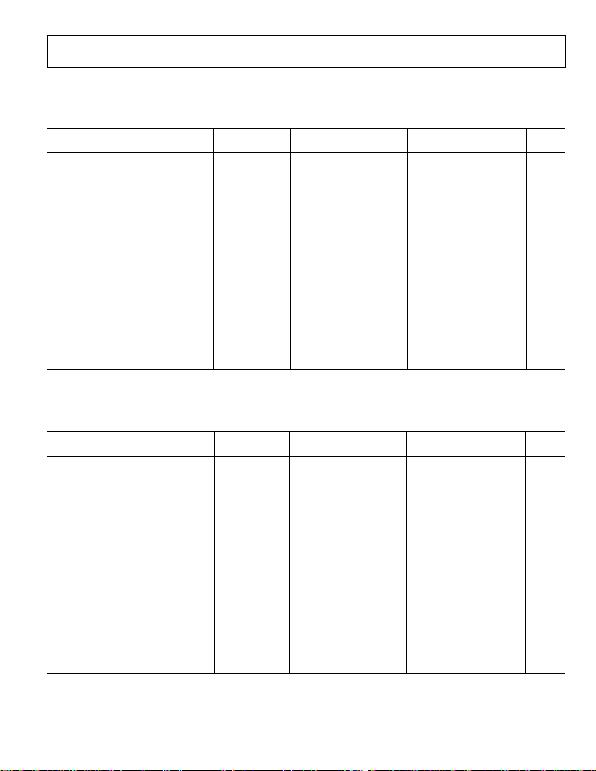Rev. B | Page 5 of 28
VS+ = +15 V, VS− = −15 V, VREF = 0 V, TA = 25°C, G = 1, RL = 2 k1, unless otherwise noted. Table 3 displays the specifications for the
dynamic performance of each individual instrumentation amplifier.
Table 3. Dynamic Performance of Each Individual Amplifier—Single-Ended Output Configuration, VS = ±15 V
Parameter Conditions Min Typ Max Min Typ Max Unit
DYNAMIC RESPONSE
Small Signal Bandwidth −3 dB
G = 1 1500 1500 kHz
G = 10 800 800 kHz
G = 100 120 120 kHz
G =1000 14 14 kHz
Settling Time 0.01% ΔVO = ±10 V step
G = 1 5 5 μs
G = 10 4.3 4.3 μs
G = 100 8.1 8.1 μs
G =1000 58 58 μs
Settling Time 0.001% ΔVO = ±10 V step
G = 1 6 6 μs
G = 10 4.6 4.6 μs
G = 100 9.6 9.6 μs
G =1000 74 74 μs
Slew Rate
G = 1 to 100 2 2 V/μs
1 When the output sinks more than 4 mA, use a 47 pF capacitor in parallel with the load to prevent ringing. Otherwise, use a larger load, such as 10 kΩ.
VS+ = +15 V, VS− = −15 V, VREF = 0 V, TA = 25°C, G = 1, RL = 2 k1, unless otherwise noted. Table 4 displays the specifications for the
dynamic performance of both amplifiers when used in the differential output configuration shown in Figure 63.
Table 4. Dynamic Performance of Both Amplifiers—Differential Output Configuration2, VS = ±15 V
Parameter Conditions Min Typ Max Min Typ Max Unit
DYNAMIC RESPONSE
Small Signal Bandwidth −3 dB
G = 1 1500 1500 kHz
G = 10 800 800 kHz
G = 100 120 120 kHz
G =1000 14 14 kHz
Settling Time 0.01% ΔVO = ±10 V step
G = 1 5 5 μs
G = 10 4.3 4.3 μs
G = 100 8.1 8.1 μs
G =1000 58 58 μs
Settling Time 0.001% ΔVO = ±10 V step
G = 1 6 6 μs
G = 10 4.6 4.6 μs
G = 100 9.6 9.6 μs
G =1000 74 74 μs
Slew Rate
G = 1 to 100 2 2 V/μs
1 When the output sinks more than 4 mA, use a 47 pF capacitor in parallel with the load to prevent ringing. Otherwise, use a larger load, such as 10 kΩ.
2 Refers to the differential configuration shown in . Figure 63Rev. B | Page 6 of 28
VS + = 5 V, VS− = 0 V, VREF = 2.5 V, TA = 25°C, G = 1, RL = 2 k1, unless otherwise noted. Table 5 displays the specifications for an
individual instrumentation amplifier configured for a single-ended output or dual instrumentation amplifiers configured for differential
outputs as shown in Figure 63.
Table 5. Individual Amplifier in Single-Ended Configuration or Dual Amplifiers in Differential Output Configuration2, VS =+5 V
Parameter Test Conditions Min Typ Max Min Typ Max Unit
COMMON-MODE REJECTION RATIO (CMRR)
CMRR DC to 60 Hz with
1 kΩ Source Imbalance
VCM = 0 to 2.5 V
G = 1 78 86 dB
G = 10 94 100 dB
G = 100 94 100 dB
G = 1000 94 100 dB
CMRR at 10 kHz
G = 1 74 80 dB
G = 10 84 90 dB
G = 100 84 90 dB
G = 1000 84 90 dB
NOISE RTI noise = √(eni2 + (eno/G)2)
Voltage Noise, 1 kHz VS = ±2.5 V
Input Voltage Noise, eni V
IN+, VIN= 0 V, VREF = 0 V 14 14 17 nV/√Hz
Output Voltage Noise, eno V
IN+, VIN= 0 V, VREF = 0 V 90 90 100 nV/√Hz
RTI, 0.1 Hz to 10 Hz
G = 1 5 5 μV p-p
G = 1000 0.8 0.8 μV p-p
Current Noise f = 1 kHz 1 1 fA/√Hz
VOLTAGE OFFSET RTI VOS = (VOSI) + (VOSO/G)
Input Offset, VOSI 300 250 μV
Average TC T = −40°C to +85°C 10 5 μV/°C
Output Offset, VOSO 1200 800 μV
Average TC T = −40°C to +85°C 10 5 μV/°C
Offset RTI vs. Supply (PSR)
G = 1 86 86 dB
G = 10 96 100 dB
G = 100 96 100 dB
G = 1000 96 100 dB
INPUT CURRENT (PER CHANNEL)
Input Bias Current 25 10 pA
Over Temperature3 T = −40°C to +85°C 300 300 pA
Input Offset Current 2 0.6 pA
Over Temperature3 T = −40°C to +85°C 5 5 pA
REFERENCE INPUT
RIN 40 40
IIN V
IN+, VIN− = 0 V 70 70 μA
Voltage Range −VS +VS −VS +VS V
Gain to Output 1 ±
0.0001
1 ±
0.0001
V/V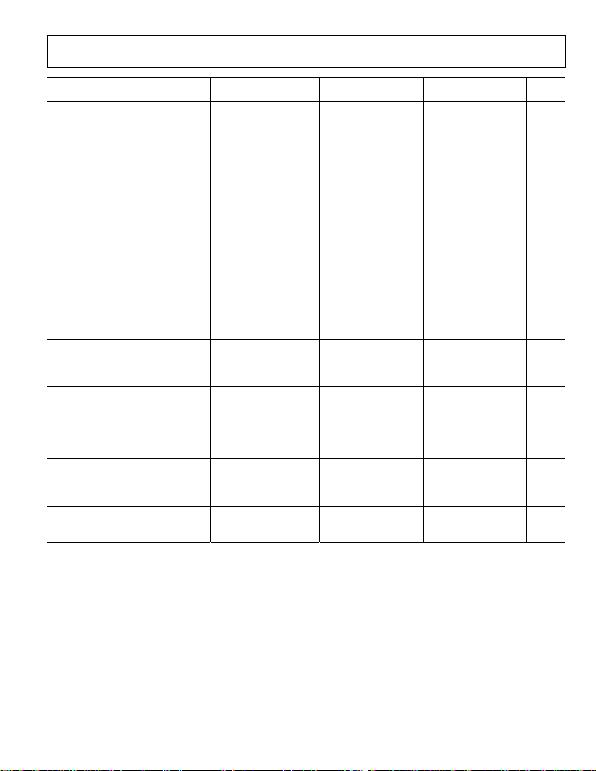Rev. B | Page 7 of 28
Parameter Test Conditions Min Typ Max Min Typ Max Unit
GAIN G = 1 + (49.4 kΩ/RG)
Gain Range 1 1000 1 1000 V/V
Gain Error
G = 1 VOUT = 0.3 V to 2.9 V 0.06 0.04 %
G = 10 VOUT = 0.3 V to 3.8 V 0.3 0.2 %
G = 100 VOUT = 0.3 V to 3.8 V 0.3 0.2 %
G = 1000 VOUT = 0.3 V to 3.8 V 0.3 0.2 %
Nonlinearity VOUT = 0.3 V to 2.9 V for G = 1
V
OUT = 0.3 V to 3.8 V for G > 1
G = 1 RL = 10 kΩ 35 50 35 50 ppm
G = 10 RL = 10 kΩ 35 50 35 50 ppm
G = 100 RL = 10 kΩ 50 75 50 75 ppm
G = 1000 RL = 10 kΩ 90 115 90 115 ppm
G = 1 RL = 2 kΩ 35 50 35 50 ppm
G = 10 RL = 2 kΩ 35 50 35 50 ppm
G = 100 RL = 2 kΩ 50 75 50 75 ppm
G = 1000 RL = 2 kΩ 175 200 175 200 ppm
Gain vs. Temperature
G = 1 3 10 2 5 ppm/°C
G > 10 −50 −50 ppm/°C
INPUT
Impedance (Pin to Ground)4 104||6 104||6 GΩ||pF
Input Voltage Range5 −0.1 +VS − 2 −0.1 +VS − 2 V
Over Temperature T = −40°C to +85°C −0.1 +VS − 2.1 −0.1 +VS − 2.1 V
OUTPUT
Output Swing RL = 2 kΩ 0.25 4.75 0.25 4.75 V
Over Temperature T = −40°C to +85°C 0.3 4.70 0.3 4.70 V
Output Swing RL = 10 kΩ 0.15 4.85 0.15 4.85 V
Over Temperature T = −40°C to +85°C 0.2 4.80 0.2 4.80 V
Short-Circuit Current 15 15 mA
POWER SUPPLY (PER AMPLIFIER)
Operating Range 4.5 36 4.5 36 V
Quiescent Current 750 800 750 800 μA
Over Temperature T = −40°C to +85°C 850 900 850 900 μA
TEMPERATURE RANGE
For Specified Performance −40 +85 −40 +85 °C
Operational6 −40 +125 −40 +125 °C
1 When the output sinks more than 4 mA, use a 47 pF capacitor in parallel with the load to prevent ringing. Otherwise, use a larger load, such as 10 kΩ.
2 Refers to the differential configuration shown in . Figure 63
3 Refer to and for the relationship between input current and temperature. Figure 14 Figure 15
4 Differential and common-mode impedance can be calculated from the pin impedance: ZDIFF = 2(ZPIN); ZCM = ZPIN/2.
5 The AD8224 can operate up to a diode drop below the negative supply, but the bias current increases sharply. The input voltage range reflects the maximum
allowable voltage where the input bias current is within the specification.
6 The AD8224 is characterized from −40°C to +125°C. See the section for expected operation in that temperature range. Typical Performance Characteristics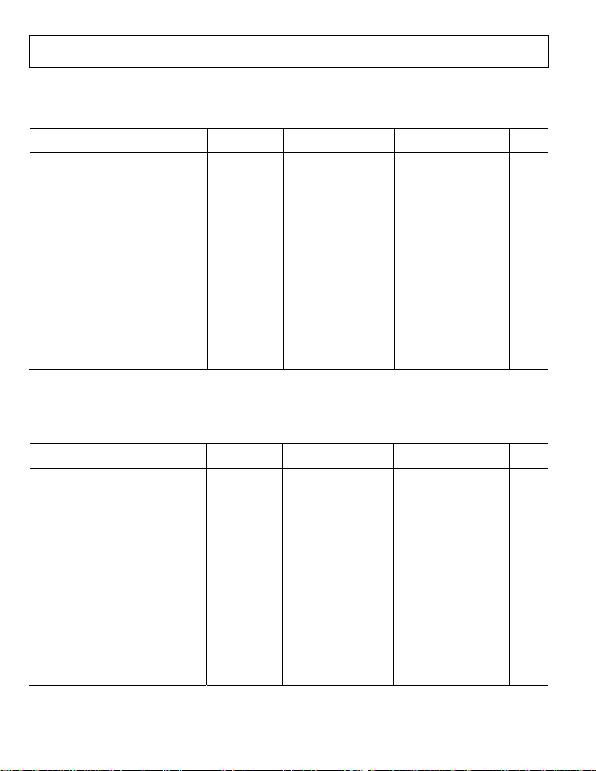Rev. B | Page 8 of 28
VS + = 5 V, VS− = 0 V, VREF = 2.5 V, TA = 25°C, G = 1, RL = 2 k1, unless otherwise noted. Table 6 displays the specifications for the
dynamic performance of each individual instrumentation amplifier.
Table 6. Dynamic Performance of Each Individual Amplifier—Single-Ended Output Configuration, VS = +5 V
Parameter Conditions Min Typ Max Min Typ Max Unit
DYNAMIC RESPONSE
Small Signal Bandwidth −3 dB
G = 1 1500 1500 kHz
G = 10 800 800 kHz
G = 100 120 120 kHz
G =1000 14 14 kHz
Settling Time 0.01%
G = 1 ΔVO = 3 V step 2.5 2.5 μs
G = 10 ΔVO = 4 V step 2.5 2.5 μs
G = 100 ΔVO = 4 V step 7.5 7.5 μs
G =1000 ΔVO = 4 V step 60 60 μs
Settling Time 0.001%
G = 1 ΔVO = 3 V step 3.5 3.5 μs
G = 10 ΔVO = 4 V step 3.5 3.5 μs
G = 100 ΔVO = 4 V step 8.5 8.5 μs
G =1000 ΔVO = 4 V step 75 75 μs
Slew Rate
G = 1 to 100 2 2 V/μs
1 When the output sinks more than 4 mA, use a 47 pF capacitor in parallel with the load to prevent ringing. Otherwise, use a larger load, such as 10 kΩ.
VS + = 5 V, VS− = 0 V, VREF = 2.5 V, TA = 25°C, G = 1, RL = 2 k1 unless otherwise noted. Table 7 displays the specifications for the
dynamic performance of both amplifiers when used in the differential output configuration shown in Figure 63.
Table 7. Dynamic Performance of Both Amplifiers—Differential Output Configuration2, VS = +5 V
Parameter Conditions Min Typ Max Min Typ Max Unit
DYNAMIC RESPONSE
Small Signal Bandwidth −3 dB
G = 1 1500 1500 kHz
G = 10 800 800 kHz
G = 100 120 120 kHz
G =1000 14 14 kHz
Settling Time 0.01%
G = 1 ΔVO = 3 V step 2.5 2.5 μs
G = 10 ΔVO = 4 V step 2.5 2.5 μs
G = 100 ΔVO = 4 V step 7.5 7.5 μs
G =1000 ΔVO = 4 V step 60 60 μs
Settling Time 0.001%
G = 1 ΔVO = 3 V step 3.5 3.5 μs
G = 10 ΔVO = 4 V step 3.5 3.5 μs
G = 100 ΔVO = 4 V step 8.5 8.5 μs
G =1000 ΔVO = 4 V step 75 75 μs
Slew Rate
G = 1 to 100 2 2 V/μs
1 When the output sinks more than 4 mA, use a 47 pF capacitor in parallel with the load to prevent ringing. Otherwise, use a larger load, such as 10 kΩ.
2 Refers to the differential configuration shown in . Figure 63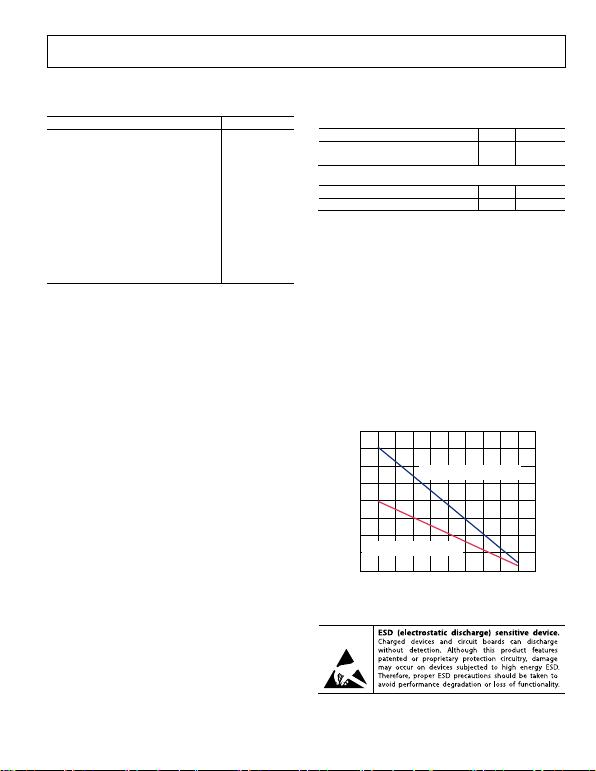Rev. B | Page 9 of 28
ABSOLUTE MAXIMUM RATINGS
Table 8.
Parameter Rating
Supply Voltage ±18 V
Power Dissipation See Figure 2
Output Short-Circuit Current Indefinite1
Input Voltage (Common Mode) ±VS
Differential Input Voltage ±VS
Storage Temperature Range −65°C to +130°C
Operating Temperature Range2 −40°C to +125°C
Lead Temperature (Soldering, 10 sec) 300°C
Junction Temperature 130°C
Package Glass Transition Temperature 130°C
ESD (Human Body Model) 4 kV
ESD (Charge Device Model) 1 kV
ESD (Machine Model) 0.4 kV
Stresses above those listed under Absolute Maximum Ratings
may cause permanent damage to the device. This is a stress
rating only; functional operation of the device at these or any
other conditions above those indicated in the operational
section of this specification is not implied. Exposure to absolute
maximum rating conditions for extended periods may affect
device reliability.
1 Assumes the load is referenced to midsupply.
2 Temperature for 85°C. For performance
to 125°C, see the section.
specified performance is −40°C to +
Typical Performance Characteristics
THERMAL RESISTANCE
Table 9.
CP-16-13: LFCSP Soldered to Board 48 °C/W
CP-16-13: LFCSP Not Soldered to Board 86 °C/W
Table 10.
CP-16-19: LFCSP 86 °C/W
The θJA values in Table 9 and Table 10 assume a 4-layer JEDEC
standard board. If the thermal pad is soldered to the board, it is
also assumed it is connected to a plane. θJC at the exposed pad is
4.4°C/W.
Maximum Power Dissipation
The maximum safe power dissipation for the AD8224 is limited
by the associated rise in junction temperature (TJ) on the die. At
approximately 130°C, which is the glass transition temperature,
the plastic changes its properties. Even temporarily exceeding
this temperature limit may change the stresses that the package
exerts on the die, permanently shifting the parametric performance
of the amplifiers. Exceeding a temperature of 130°C for an
extended period can result in a loss of functionality. Figure 2
shows the maximum safe power dissipation in the package vs.
the ambient temperature for the LFCSP on a 4-layer JEDEC
standard board.
0
0.5
1.0
1.5
2.0
2.5
3.0
3.5
4.0
–60 –40 –20 0 20 40 60 80 100 120 140
06286-002
AMBIENT TEMPERATURE (°C)
MAXIMUM POWER DISSIPATION (W)
JA
IS SOLDERED TO BOARD
JA
IS NOT SOLDERED TO BOARD
Figure 2. Maximum Power Dissipation vs. Ambient Temperature
ESD CAUTION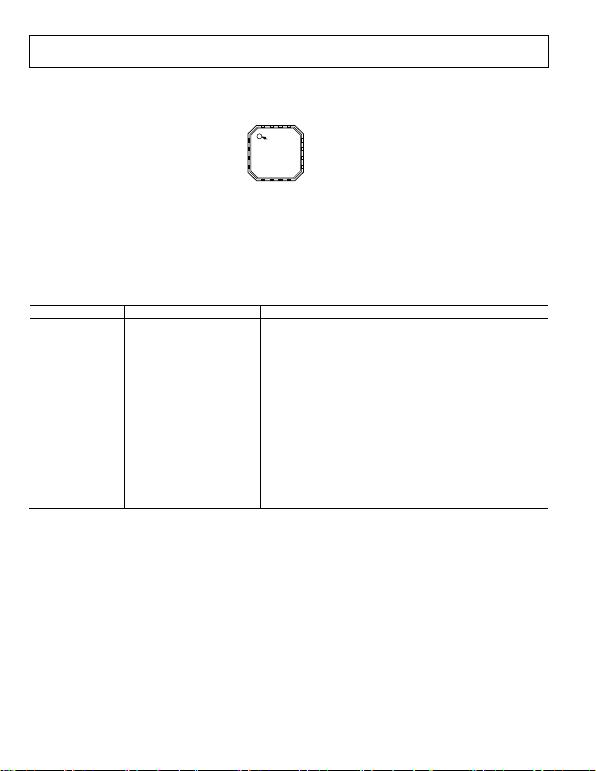Rev. B | Page 10 of 28
PIN CONFIGURATION AND FUNCTION DESCRIPTIONS
06286-003
12
11
10
9
–IN1 1
R
G1
2
R
G1
3
+V
S
5
REF1 6
REF2 7
–V
S
8
+IN1 4
16
15
14
13
PIN 1
INDICATOR
TOP VIEW
–IN2
R
G2
R
G2
+IN2
+V
S
OUT1
OUT2
–V
S
NOTES
1. THE AD8224 COMES IN TWO PACKAGE TYPES—EACH IS A 16 LEAD
4mm × 4mm LFCSP. ONE PACKAGE HAS AN EXPOSED THERMAL PAD,
WHICH IS CONNECTED TO +VS. THE OTHER PACKAGE TYPE DOES NOT
EXPOSE THE THERMAL PAD. SEE THE PACKAGE CONSIDERATIONS
Figure 3. Pin Configuration
Table 11. Pin Function Descriptions
Pin Number Mnemonic Description
1 −IN1 Negative Input Instrumentation Amplifier (In-Amp) 1
2 RG1 Gain Resistor In-Amp 1
3 RG1 Gain Resistor In-Amp 1
4 +IN1 Positive Input In-Amp 1
5 +VS Positive Supply
6 REF1 Reference Adjust In-Amp 1
7 REF2 Reference Adjust In-Amp 2
8 −VS Negative Supply
9 +IN2 Positive Input In-Amp 2
10 RG2 Gain Resistor In-Amp 2
11 RG2 Gain Resistor In-Amp 2
12 −IN2 Negative Input In-Amp 2
13 −VS Negative Supply
14 OUT2 Output In-Amp 2
15 OUT1 Output In-Amp 1
16 +VS Positive Supply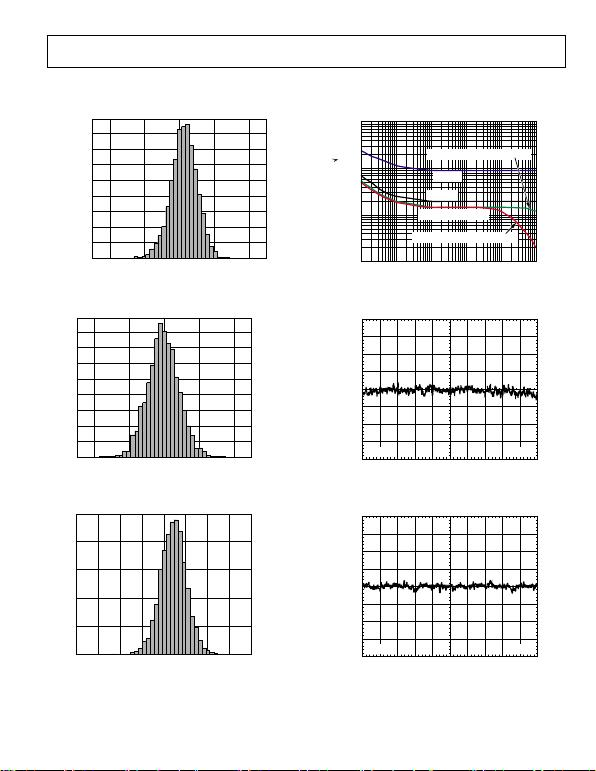Rev. B | Page 11 of 28
TYPICAL PERFORMANCE CHARACTERISTICS
25°C, VS = ±15 V, RL =10 kΩ, unless otherwise noted.
06286-070
0
CMRR (µV/V)
NUMBER OF UNITS
–40 –20 0 20 40
400
350
300
250
200
150
100
50
Figure 4. Typical Distribution of CMRR (G = 1)
06286-071
0
V
OSI
(µV)
NUMBER OF UNITS
–200 –100 0 100 200
400
350
300
250
200
150
100
50
Figure 5. Typical Distribution of Input Offset Voltage
06286-072
400
300
200
100
0
–1200 –900 –600 –300 0 300 600 900 1200
VOSO (µV)
NUMBER OF UNITS
Figure 6. Typical Distribution of Output Offset Voltage
1000
1
1 100k
FREQUENCY (Hz)
VOLTAGE NOISE RTI (nV/ Hz)
10 100 1k 10k
10
100
GAIN = 100 BANDWIDTH ROLL-OFF
GAIN = 1
GAIN = 10
GAIN = 100/GAIN = 1000
GAIN = 1000 BANDWIDTH ROLL-OFF
06286-009
Figure 7. Voltage Spectral Density vs. Frequency
X
X
XX
XX XX
XXX (X)
XXX (X)
1s/DIV5µV/DIV
06286-010
Figure 8. 0.1 Hz to 10 Hz RTI Voltage Noise (G = 1)
X
X
XX
XX XX
XXX (X)
XXX (X)
1s/DIV1µV/DIV
06286-011
Figure 9. 0.1 Hz to 10 Hz RTI Voltage Noise (G = 1000)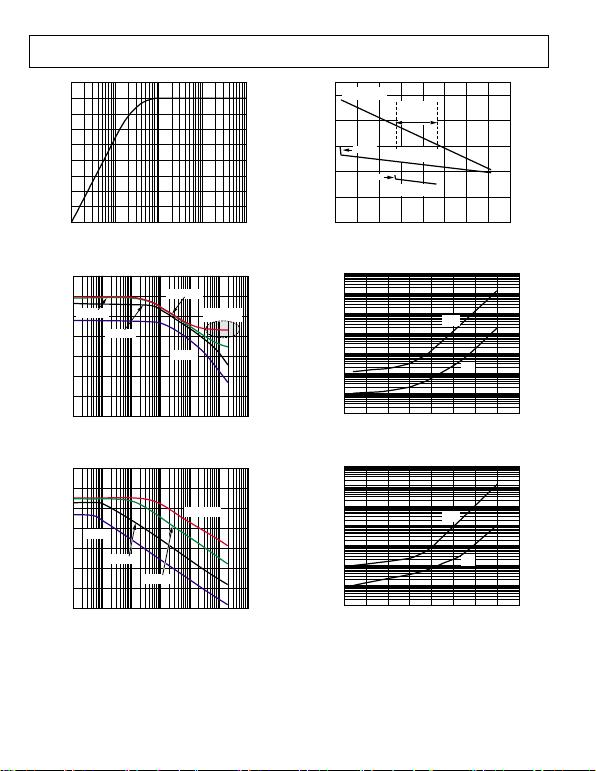Rev. B | Page 12 of 28
0
0.5
1.0
1.5
2.0
2.5
3.0
3.5
4.0
4.5
0.1 1 10 100 1000
06286-012
TIME (s)
DELTA V
OSI
(µV)
Figure 10. Change in Input Offset Voltage vs. Warmup Time
150
10
11M
FREQUENCY (Hz)
PSRR (dB)
10 100 1k 10k 100k
130
110
90
70
50
30
GAIN = 1000
BANDWIDTH
LIMITED
GAIN = 100
GAIN = 10
GAIN = 1
06286-013
Figure 11. Positive PSRR vs. Frequency, RTI
150
10
11
FREQUENCY (Hz)
PSRR (dB)
M
10 100 1k 10k 100k
130
110
90
70
50
30
GAIN = 1
GAIN = 10
GAIN = 100
GAIN = 1000
06286-014
Figure 12. Negative PSRR vs. Frequency, RTI
0
6286-068
COMMON-MODE VOLTAGE (V)
INPUT BIAS CURRENT (pA)
–1
1
3
5
7
9
–16 –12 –8 –4 0 4 8 12 16
–0.5
–0.3
–0.1
0.1
0.3
INPUT OFFSET CURRENT (pA)
–15.1V INPUT BIAS
CURRENT ±15
INPUT
OFFSET
CURRENT ±5
INPUT BIAS
CURRENT ±5
–5.1V
INPUT OFFSET
CURRENT ±15
Figure 13. Input Bias Current and Input Offset Current vs. Common-Mode Voltage
10n
1n
100p
10p
1p
0.1p
–50 150
TEMPERATUREC)
INPUT BIAS CURRENT (A)
–25 0 25 50 75 100 125
I
OS
I
BIAS
06286-016
Figure 14. Input Bias Current and Offset Current vs. Temperature,
VS = ±15 V, VREF = 0 V
10n
1n
100p
10p
1p
0.1p
–50 150
TEMPERATUREC)
CURRENT (A)
–25 0 25 50 75 100 125
I
OS
I
BIAS
06286-017
Figure 15. Input Bias Current and Offset Current vs. Temperature,
VS = 5 V, VREF = 2.5 V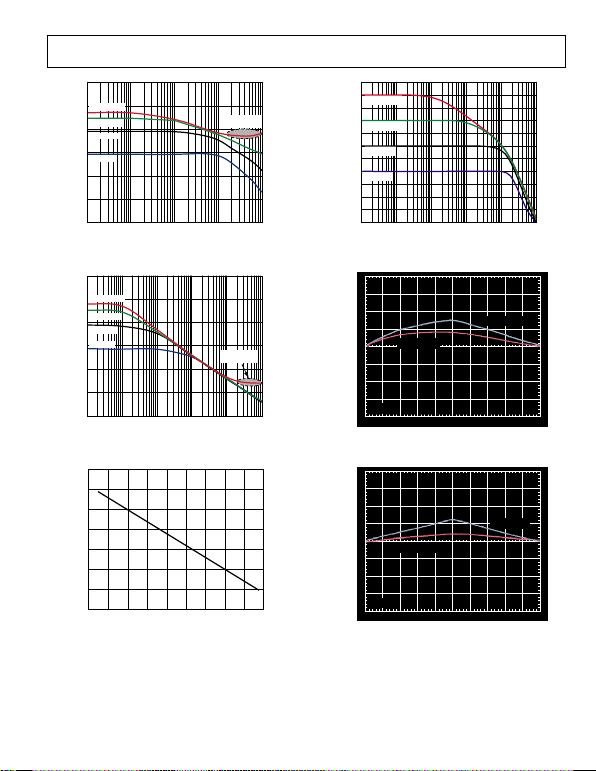Rev. B | Page 13 of 28
40
60
80
100
120
140
160
10 100 1000 10000 100000
GAIN = 1000
GAIN = 100
GAIN = 10
GAIN = 1
06286-018
FREQUENCY (Hz)
CMRR (dB)
BANDWIDTH
LIMITED
Figure 16. CMRR vs. Frequency
40
60
80
100
120
140
160
1 10 100 1000 10000 100000
GAIN = 1000
GAIN = 100
GAIN = 1
GAIN = 10
06286-019
FREQUENCY (Hz)
CMRR (dB)
BANDWIDTH
LIMITED
Figure 17. CMRR vs. Frequency, 1 kΩ Source Imbalance
0
1
3
5
2
4
6
7
–50 –30 –10 10 30 50 70 90 110 130
06286-020
TEMPERATURE (°C)
CMRR (µV/V)
Figure 18. Change in CMRR vs. Temperature, G = 1
70
–40
100 10M
FREQUENCY (Hz)
GAIN (dB)
1k 10k 100k 1M
60
50
40
30
20
10
0
–10
–20
–30
GAIN = 1
GAIN = 10
GAIN = 100
GAIN = 1000
06286-021
Figure 19. Gain vs. Frequency
OUTPUT VOLTAGE (V)
XXX
NONLINEARITY (10ppm/DIV)
–8–10 –6 –4 –2 0 2 4 6 8 10
R
= 2k
R
= 10k
V
S
= ±15V
06286-022
Figure 20. Gain Nonlinearity, G = 1
OUTPUT VOLTAGE (V)
XXX
NONLINEARITY (10ppm/DIV)
–8–10 –6 –4 –2 0 2 4 6 8 10
R
= 2k
R
= 10k
V
S
= ±15V
06286-023
Figure 21. Gain Nonlinearity, G = 10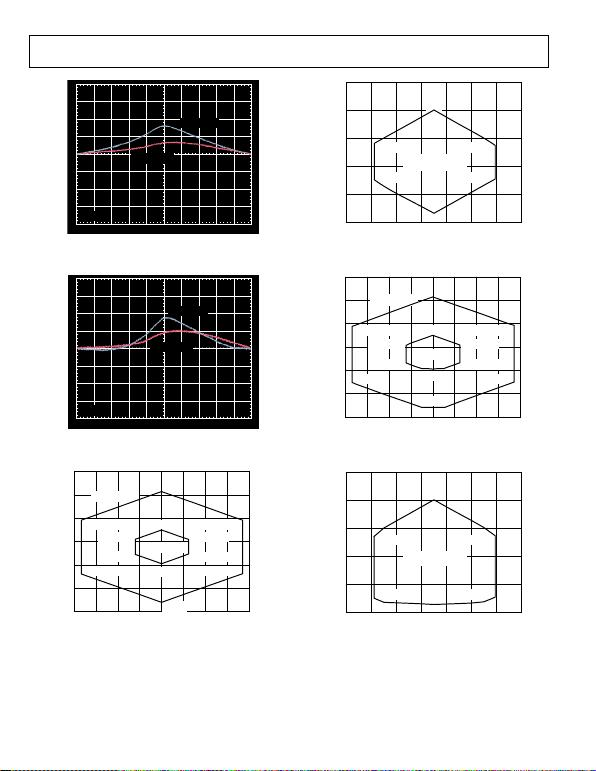Rev. B | Page 14 of 28
OUTPUT VOLTAGE (V)
XXX
NONLINEARITY (20ppm/DIV)
–8–10 –6 –4 –2 0 2 4 6 8 10
R
= 2k
R
= 10k
V
S
= ±15V
06286-024
Figure 22. Gain Nonlinearity, G = 100
OUTPUT VOLTAGE (V)
XXX
NONLINEARITY (100ppm/DIV)
–8–10 –6 –4 –2 0 2 4 6 8 10
R
= 2k
R
= 10k
V
S
= ±15V
06286-025
Figure 23. Gain Nonlinearity, G = 1000
18
–18
–16 16
OUTPUT VOLTAGE (V)
INPUT COMMON-MODE VOLTAGE (V)
12
6
0
–6
–12
–12 –8 –4 0 4 8 12
–15.3V
+13V
±15V SUPPLIES
±5V SUPPLIES
+14.9V, –8.3V
+14.9V, +5.5V
+4.95V, –3.3V
+4.95V, +0.6V
–14.8V, +5.5V
–14.8V, –8.3V
–4.8V, –3.3V
–4.8V, +0.6V
–5.3V
+3V
06286-026
Figure 24. Input Common-Mode Voltage Range vs. Output Voltage,
G = 1, VREF = 0 V
012345
4
–1
–1 6
OUTPUT VOLTAGE (V)
INPUT COMMON-MODE VOLTAGE (V)
3
2
1
0
–0.3V
+3V
+4.9V, +0.5V
+4.9V, +1.7V
+5V SINGLE SUPPLY,
V
REF
= +2.5V
+0.1V, +0.5V
+0.1V, +1.7V
06286-027
Figure 25. Input Common-Mode Voltage Range vs. Output Voltage,
G = 1, VS = 5 V, VREF = 2.5 V
18
–18
–16 16
OUTPUT VOLTAGE (V)
INPUT COMMON-MODE VOLTAGE (V)
12
6
0
–6
–12
–12 –8 –4 0 4 8 12
+13V
–15.3V
±15V SUPPLIES
+14.9V, –9V
+14.9V, +5.4V
+4.9V, +0.5V
+4.9V, –4.1V
–4.9V, +0.4V
–4.9V, –4.1V
–5.3V
+3V
–14.8V, –9V
–14.9V, +5.4V
±5V SUPPLIES
06286-028
Figure 26. Input Common-Mode Voltage Range vs. Output Voltage,
G = 100, VREF = 0 V
012345
4
–1
–1 6
OUTPUT VOLTAGE (V)
INPUT COMMON-MODE VOLTAGE (V)
3
2
1
0
+3V
–0.3V
+4.9V, +1.7V
+4.9V, –0.5V
+0.1V, +1.7V
+0.1V, –0.5V
+5V SINGLE SUPPLY,
V
REF
= +2.5V
06286-029
Figure 27. Input Common-Mode Voltage Range vs. Output Voltage,
G = 100, VS = 5 V, VREF = 2.5 V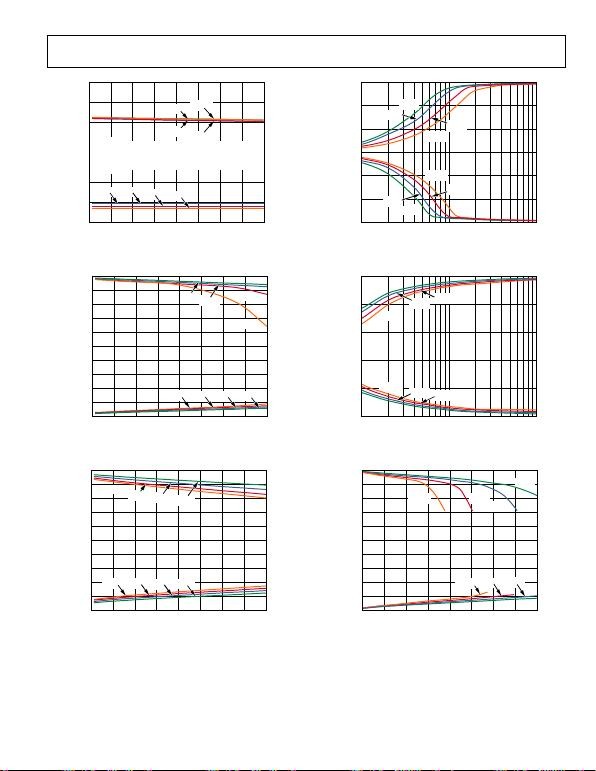Rev. B | Page 15 of 28
V
S
+
–1
21
SUPPLY VOLTAGE (V)
INPUT VOLTAGE LIMIT (V)
8
–1
–2
+1
V
S
4 6 8 10 12 14 16
–40°C +125°C
+25°C +85°C
+25°C–40°C
NOTES
1. THE AD8224 CAN OPERATE UP TO A V
BE
BELOW
THE NEGATIVE SUPPLY, BUT THE BIAS CURRENT
WILL INCREASE SHARPLY.
+85°C +125°C
06286-030
Figure 28. Input Voltage Limit vs. Supply Voltage, G = 1, VREF =0 V
V
S+
VS
21
DUAL SUPPLY VOLTAGE (±V)
OUTPUT VOLTAGE SWING (V)
REFERRED TO SUPPLY VOLTAGES
8
–1
–2
–3
–4
+4
+3
+2
+1
4 6 8 10 12 14 16
–40°C +25°C
+125°C
+85°C
–40°C
+25°C
+85°C
+125°C
06286-031
Figure 29. Output Voltage Swing vs. Dual Supply Voltage,
RLOAD = 2 kΩ, G = 10, VREF = 0 V
V
S
+
V
S
21
DUAL SUPPLY VOLTAGE (±V)
OUTPUT VOLTAGE SWING (V)
REFERRED TO SUPPLY VOLTAGES
8
–0.2
–0.4
+0.4
+0.2
4 6 8 10 12 14 16
+85°C
+125°C +25°C –40°C
–40°C
+25°C
+85°C
+125°C
06286-032
Figure 30. Output Voltage Swing vs. Dual Supply Voltage,
RLOAD = 10 kΩ, G = 10, VREF = 0 V
15
–15
100 10k
R
()
OUTPUT VOLTAGE SWING (V)
1k
10
5
0
–5
–10
+125°C
+85°C
+25°C
–40°C
+125°C
+85°C
+25°C
–40°C
06286-033
Figure 31. Output Voltage Swing vs. Load Resistance, VS = ±15 V, VREF = 0 V
5
0
100 10k
R
()
OUTPUT VOLTAGE SWING (V)
1k
4
3
2
1
–40°C
–40°C
+125°C
+125°C
+25°C
+25°C
+85°C
+85°C
06286-034
Figure 32. Output Voltage Swing vs. Load Resistance, VS = 5 V, VREF = 2.5 V
V
S
+
V
S
01
I
OUT
(mA)
OUTPUT VOLTAGE SWING (V)
REFERRED TO SUPPLY VOLTAGES
6
–1
–2
–3
–4
+4
+3
+2
+1
2 4 6 8 101214
–40°C
+125°C +85°C
+25°C
–40°C
+25°C
+85°C
+125°C
06286-035
Figure 33. Output Voltage Swing vs. Output Current, VS = ±15 V, VREF = 0 V